# RD Sharma Solutions for Class 10 Maths Chapter 3 Pair of Linear Equations in Two Variables Exercise 3.9

Age problems have always been confusing among students. Using methods of solving linear pairs of equations in two variables – often problems related to age – are solved with ease here. To know how this is done in the correct manner, refer to the RD Sharma Solutions Class 10. The students can also make use of the RD Sharma Solutions for Class 10 Maths Chapter 3 Pair of Linear Equations in Two Variables Exercise 3.9 PDF given below for any reference related to this exercise.

## RD Sharma Solutions for Class 10 Maths Chapter 3 Pair of Linear Equations in Two Variables Exercise 3.9 Download PDF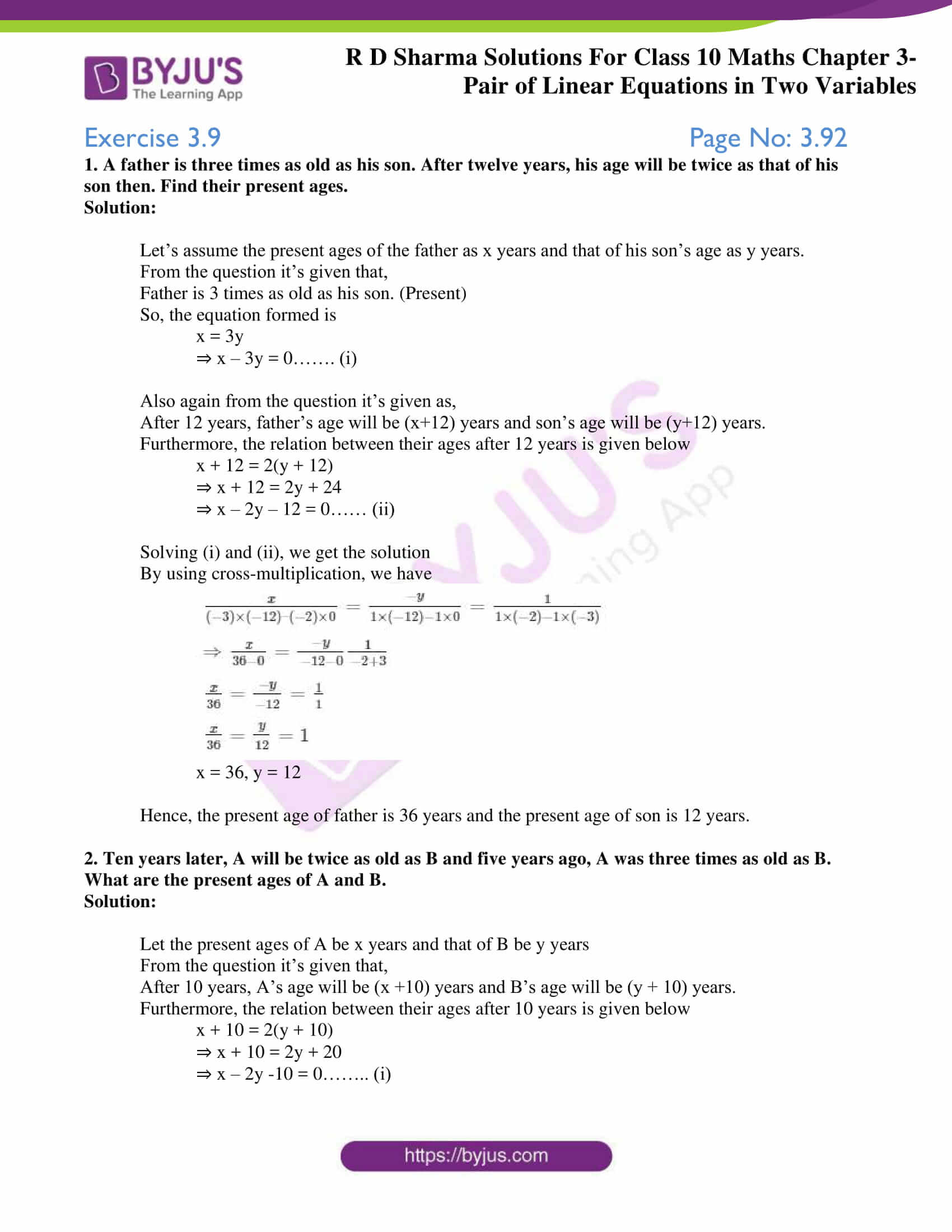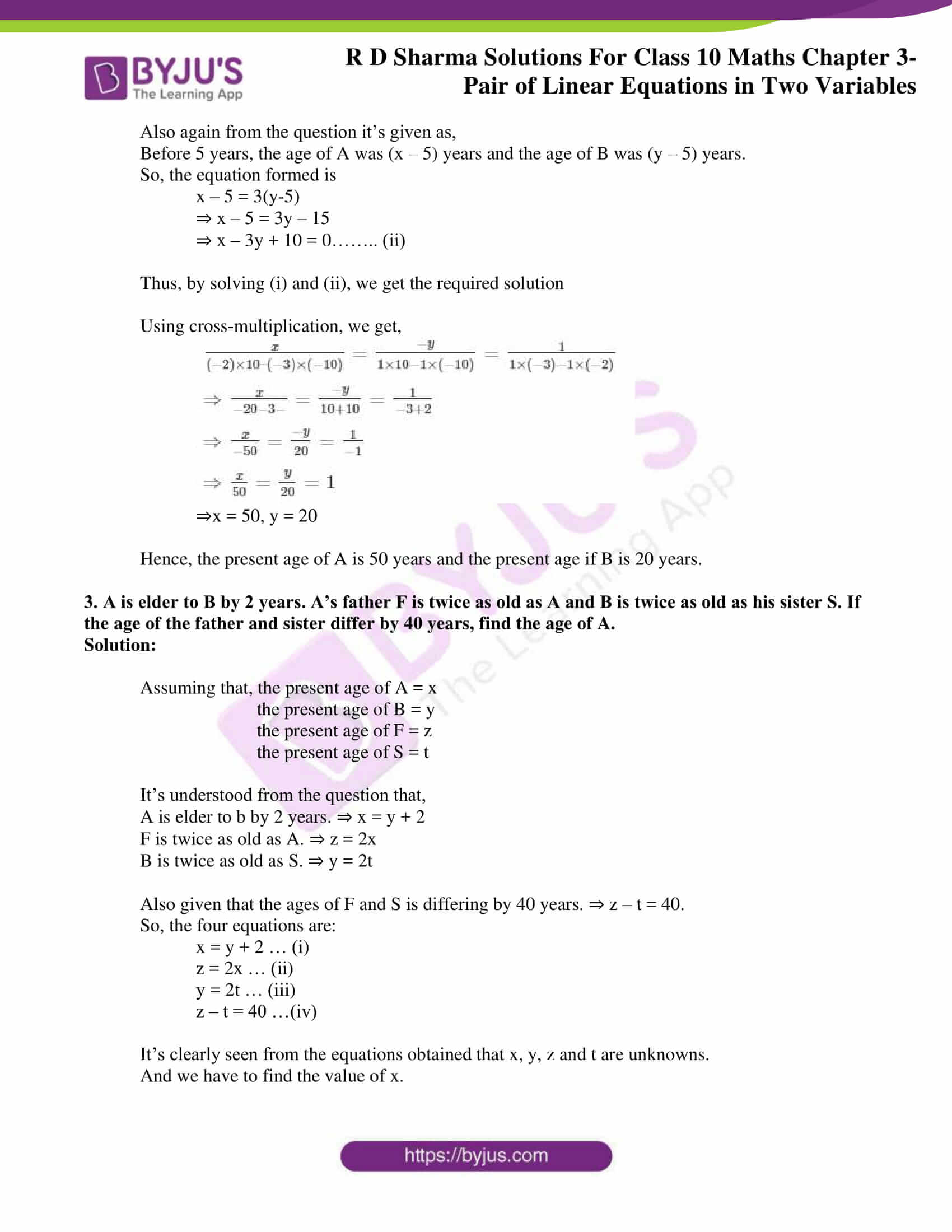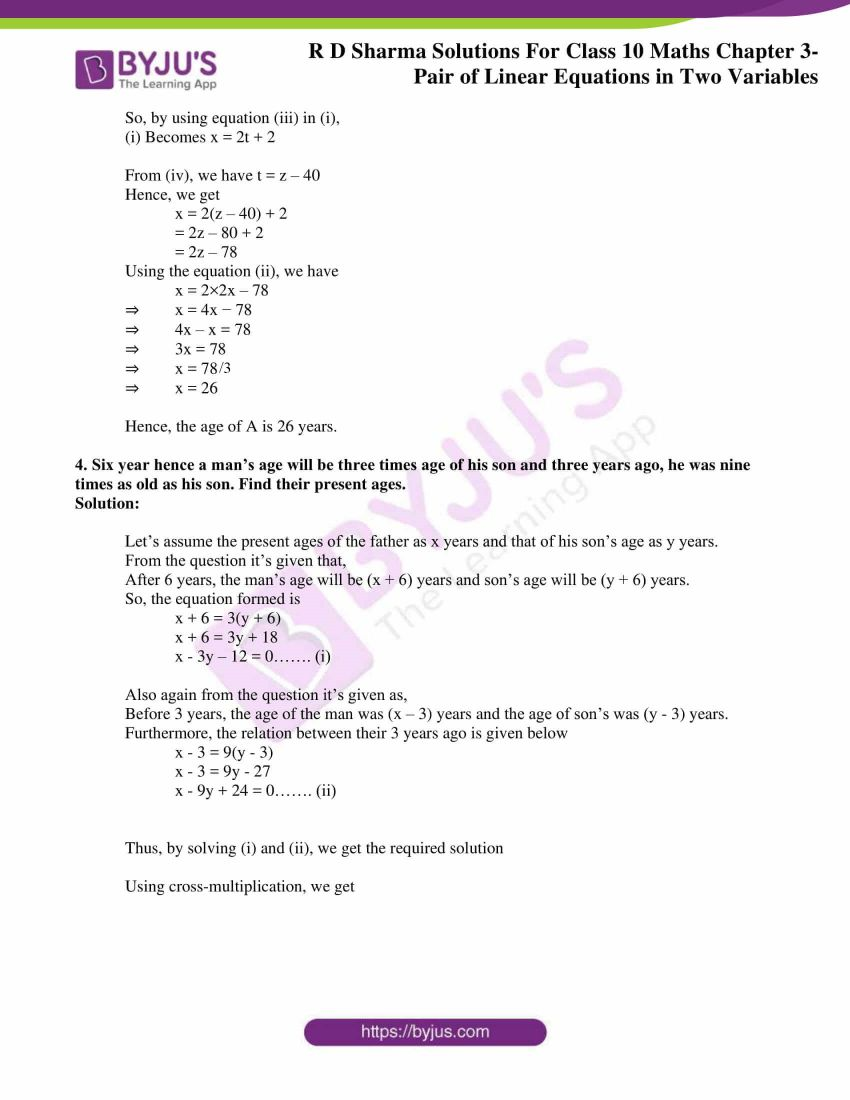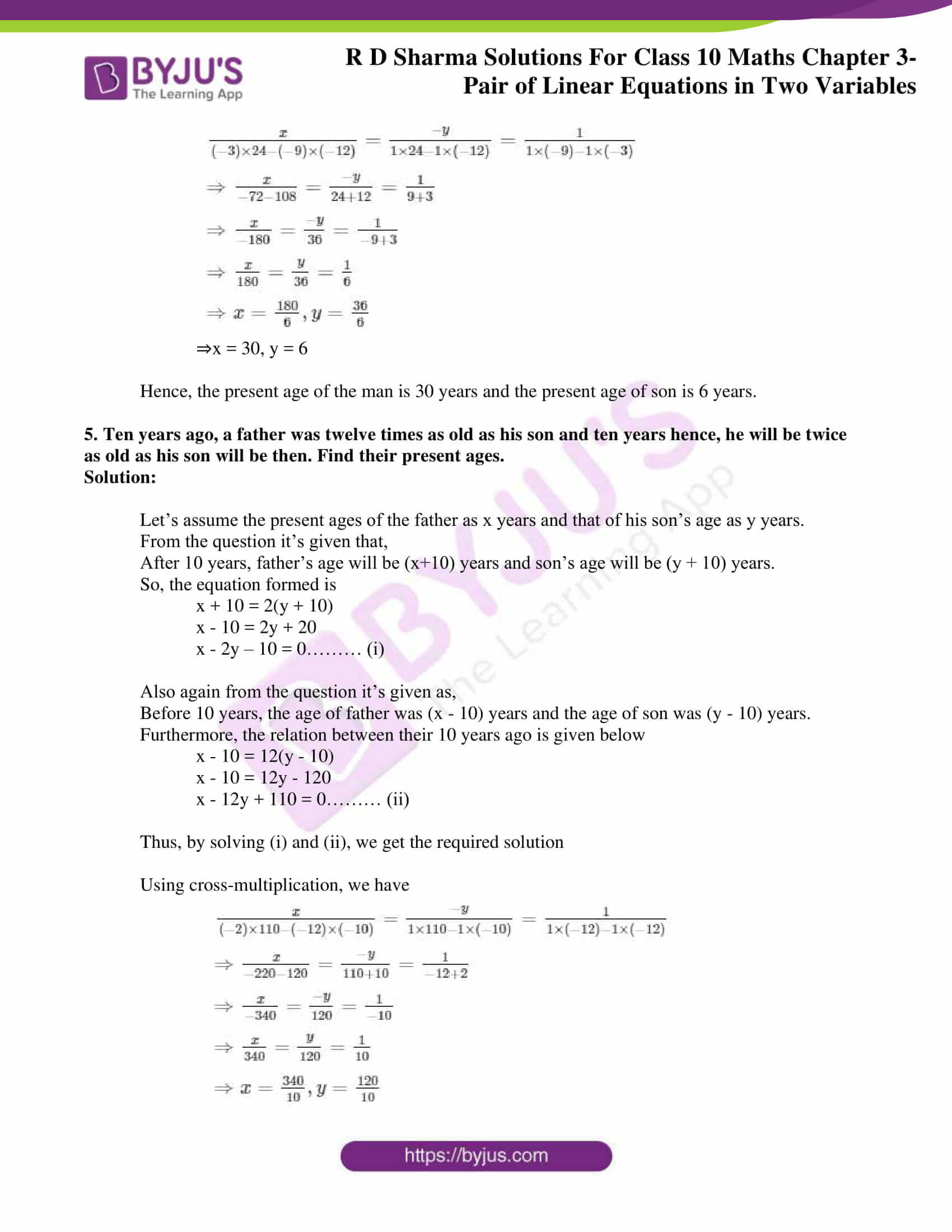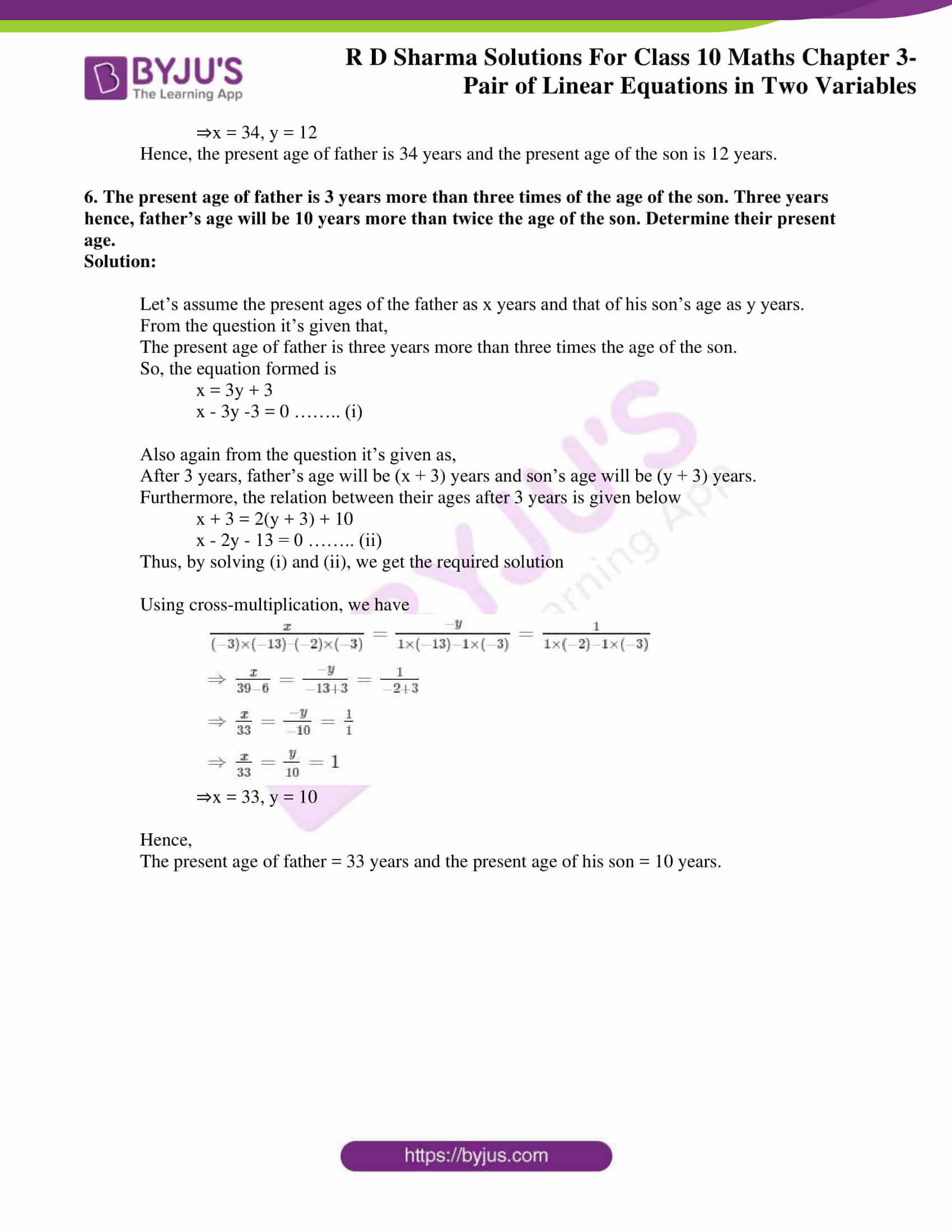### Access RD Sharma Solutions for Class 10 Maths Chapter 3 Pair of Linear Equations In Two Variables Exercise 3.9

1. A father is three times as old as his son. After twelve years, his age will be twice as that of his son then. Find their present ages.

Solution:

Let’s assume the present ages of the father as x years and that of his son’s age as y years.

From the question, it’s given that,

Father is 3 times as old as his son. (Present)

So, the equation formed is

x = 3y

⇒ x – 3y = 0……. (i)

Also, again from the question, it’s given as,

After 12 years, the father’s age will be (x+12) years and the son’s age will be (y+12) years.

Furthermore, the relation between their ages after 12 years is given below

x + 12 = 2(y + 12)

⇒ x + 12 = 2y + 24

⇒ x – 2y – 12 = 0…… (ii)

Solving (i) and (ii), we get the solution

By using cross-multiplication, we have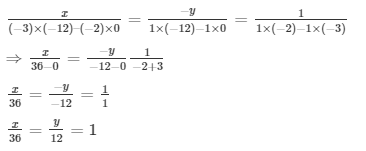x = 36, y = 12

Hence, the present age of the father is 36 years and the present age of the son is 12 years.

2. Ten years later, A will be twice as old as B and five years ago, A was three times as old as B. What are the present ages of A and B.

Solution:

Let the present ages of A be x years and that of B be y years

From the question, it’s given that,

After 10 years, A’s age will be (x +10) years and B’s age will be (y + 10) years.

Furthermore, the relation between their ages after 10 years is given below

x + 10 = 2(y + 10)

⇒ x + 10 = 2y + 20

⇒ x – 2y -10 = 0…….. (i)

Also, again from the question, it’s given as,

Before 5 years, the age of A was (x – 5) years and the age of B was (y – 5) years.

So, the equation formed is

x – 5 = 3(y-5)

⇒ x – 5 = 3y – 15

⇒ x – 3y + 10 = 0…….. (ii)

Thus, by solving (i) and (ii), we get the required solution

Using cross-multiplication, we get,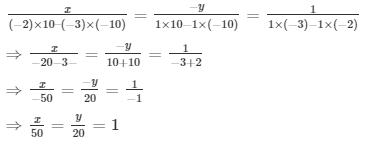⇒x = 50, y = 20

Hence, the present age of A is 50 years and the present age if B is 20 years.

3. A is elder to B by 2 years. A’s father F is twice as old as A and B is twice as old as his sister S. If the age of the father and sister differ by 40 years, find the age of A.

Solution:

Assuming that the present age of A = x

the present age of B = y

the present age of F = z

the present age of S = t

It’s understood from the question that,

A is elder than b by 2 years. ⇒ x = y + 2

F is twice as old as A. ⇒ z = 2x

B is twice as old as S. ⇒ y = 2t

Also, given that the ages of F and S differ by 40 years. ⇒ z – t = 40.

So, the four equations are:

x = y + 2 … (i)

z = 2x … (ii)

y = 2t … (iii)

z – t = 40 …(iv)

It’s clearly seen from the equations obtained that x, y, z and t are unknowns.

And we have to find the value of x.

So, by using equation (iii) in (i),

(i) Becomes x = 2t + 2

From (iv), we have t = z – 40

Hence, we get

x = 2(z – 40) + 2

= 2z – 80 + 2

= 2z – 78

Using equation (ii), we have

x = 2×2x – 78

⇒ x = 4x − 78

⇒ 4x – x = 78

⇒ 3x = 78

⇒ x = 78/3

⇒ x = 26

Hence, the age of A is 26 years.

4. Six years hence a man’s age will be three times age of his son and three years ago, he was nine times as old as his son. Find their present ages.

Solution:

Let’s assume the present ages of the father as x years and that of his son’s age as y years.

From the question, it’s given that,

After 6 years, the man’s age will be (x + 6) years and son’s age will be (y + 6) years.

So, the equation formed is

x + 6 = 3(y + 6)

x + 6 = 3y + 18

x – 3y – 12 = 0……. (i)

Also, again from the question, it’s given as,

Before 3 years, the age of the man was (x – 3) years and the age of son’s was (y – 3) years.

Furthermore, the relation between their 3 years ago is given below

x – 3 = 9(y – 3)

x – 3 = 9y – 27

x – 9y + 24 = 0……. (ii)

Thus, by solving (i) and (ii), we get the required solution

Using cross-multiplication, we get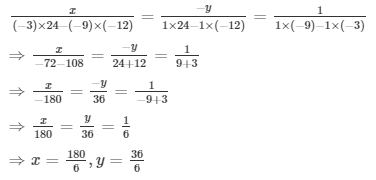⇒x = 30, y = 6

Hence, the present age of the man is 30 years and the present age of the son is 6 years.

5. Ten years ago, a father was twelve times as old as his son and ten years hence, he will be twice as old as his son will be then. Find their present ages.

Solution:

Let’s assume the present age of the father as x years and that of his son’s age as y years.

From the question, it’s given that,

After 10 years, the father’s age will be (x+10) years and the son’s age will be (y + 10) years.

So, the equation formed is

x + 10 = 2(y + 10)

x – 10 = 2y + 20

x – 2y – 10 = 0……… (i)

Also, again from the question, it’s given as,

Before 10 years, the age of the father was (x – 10) years and the age of the son was (y – 10) years.

Furthermore, the relationship between their ages 10 years ago is given below

x – 10 = 12(y – 10)

x – 10 = 12y – 120

x – 12y + 110 = 0……… (ii)

Thus, by solving (i) and (ii), we get the required solution

Using cross-multiplication, we have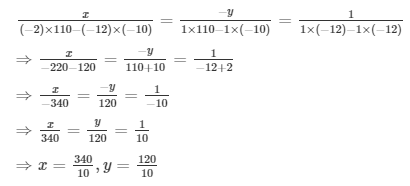⇒x = 34, y = 12

Hence, the present age of the father is 34 years and the present age of the son is 12 years.

6. The present age of the father is 3 years more than three times of the age of the son. Three years hence, the father’s age will be 10 years more than twice the age of the son. Determine their present age.

Solution:

Let’s assume the present age of the father as x years and that of his son’s age as y years.

From the question, it’s given that,

The present age of the father is three years more than three times the age of the son.

So, the equation formed is

x = 3y + 3

x – 3y -3 = 0 …….. (i)

Also, again from the question, it’s given as,

After 3 years, the father’s age will be (x + 3) years and the son’s age will be (y + 3) years.

Furthermore, the relation between their ages after 3 years is given below

x + 3 = 2(y + 3) + 10

x – 2y – 13 = 0 …….. (ii)

Thus, by solving (i) and (ii), we get the required solution

Using cross-multiplication, we have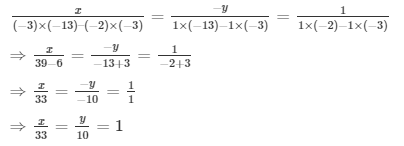⇒x = 33, y = 10

Hence,

The present age of the father = 33 years and the present age of his son = 10 years.